# Class 12 Maths NCERT Solutions for Chapter 10 Vector Algebra Exercise 10.2### Vector Algebra Exercise 10.2 Solutions

1. Compute the magnitude of the following vectors :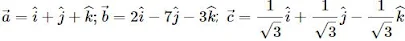Solution

The given vectors are :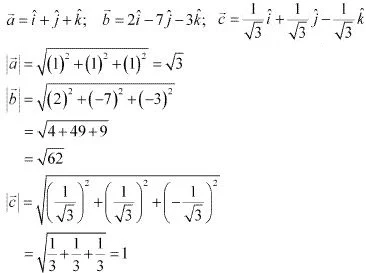2. Write two different vectors having same magnitude.

Solution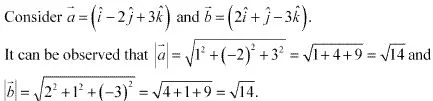Hence, a⃗ and b⃗ are two different vectors having the same magnitude. The vectors are different because they have different directions.

3. Write two different vectors having same direction.
Solution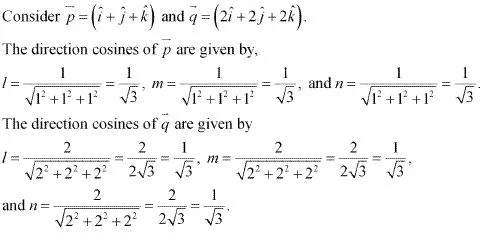The direction cosines of p⃗ and q⃗ are the same. Hence, the two vectors have the same direction.

4. Find the values of x and y so that the vectors 2i + 3j  and  xi + yj  are equal.
Solution
The two vectors 2i + 3j  and  xi + yj  will be equal if their corresponding components are equal.
Hence, the required values of x and y are 2 and 3 respectively.

5. Find the scalar and vector components of the vector with initial point (2, 1) and terminal point (–5, 7).
Solution
The vector with the initial point P(2, 1) and terminal point Q(-5,  7) can be given by,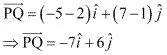Hence, the required scalar components are -7 and 6 while the vector components are -7i and  6j ^.

6. Find the sum of the vectors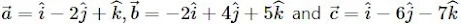Solution
The given vectors are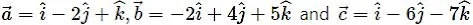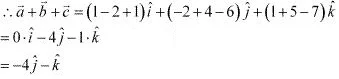7. Find the unit vector in the direction of the vector a = i + j ^  + 2k^  .
Solution
The unit vector a ^ in the direction of vector  a = i + j ^  + 2k^  is given by a ^  =  a /|a|  .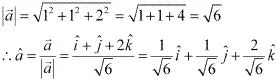8. Find the unit vector in the direction of vector PQ, where P and Q are the points (1, 2, 3) and (4, 5, 6), respectively.
Solution
The given points are P(1, 2, 3) and Q(4, 5, 6).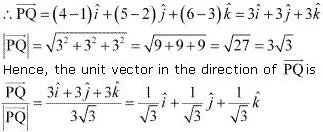Solution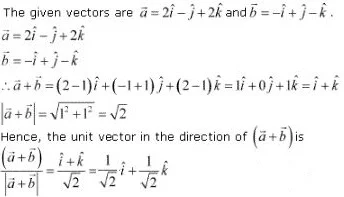10. Find a vector in the direction of vector = 5i - j ^  + 2k^  which has a magnitude of 8 units .
Solution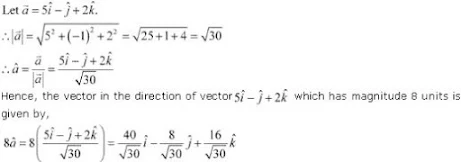11. Show that the vectors 2i - 3j ^  + 4k^ are collinear.
Solution

12. Find the direction cosines of the vector i + 2j ^  + 3k^  .

Solution13. Find the direction cosines of the vector joining the points A (1, 2, -3) and B (-1, -2, 1) directed from A to B.
Solution
The given points are A(1, 2, -3) and B(-1, -2, 1).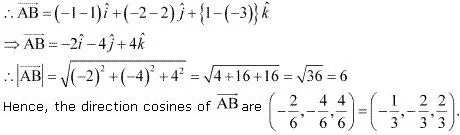14. Show that the vector  i + j ^  + k^  .is equally inclined to the axes OX, OY, and OZ.
Solution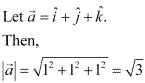Therefore, the direction cosines of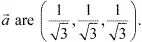Now, let α, β and γ be the angles formed by a⃗ with the positive directions of x, y, and z axes.
Then, we have cos α = 1/√3 , cosβ = 1/√3, cos γ = 1/√3 .
Hence, the given vector is equally inclined to axes OX, OY, and OZ.

15. Find the position vector of a point R which divides the line joining two points P and Q whose position vectors are i ^+ 2j ^ - k^  and -i ^+ j ^ + k^ respectively, in the ratio 2:1.
(i) Internally
(ii) Externally
Solution
The position vector of point R dividing the line segment joining two points P and Q in the ratio m : n is given by :
(i) Internally :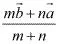(ii) Externally :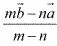Position vectors of P and Q are given as :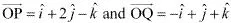(i) The position vector of point R which divides the line joining two points P and Q internally in the ratio 2: 1 is given by,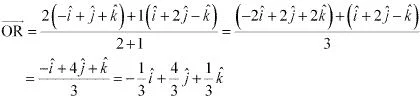(ii) The position vector of point R which divides the line joining two points P and Q externally in the ratio 2 : 1 is given by,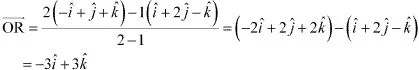16. Find the position vector of the mid point of the vector joining the points P (2, 3, 4) and Q (4, 1, –2).
Solution
The position vector of mid - point R of the vector joining points P(2, 3, 4) and Q(4, 1, -2) is given by,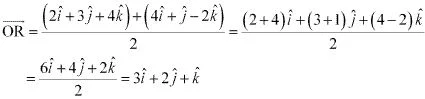17. Show that the points A , B and C with position vectors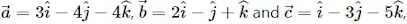respectively form the vertices of a right angled triangle.
Solution
Position vectors of points A, B, and C are respectively given as :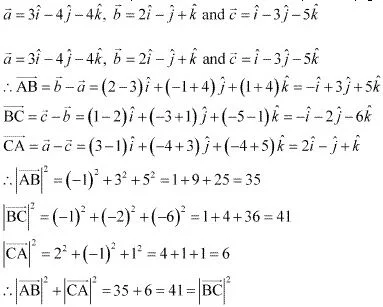Hence, ABC is a right - angled triangle.

18. In triangle ABC which of the following is not true: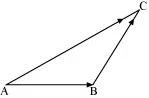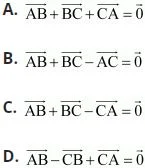Solution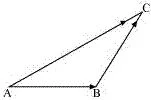On applying the triangle law of addition in the given triangle, we have :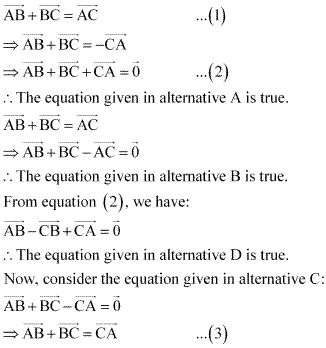From equations (1) and (3), we have :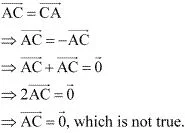Hence, the equation given in alternative C is incorrect.

19. If  a⃗ and b⃗ are two collinear vectors, then which of the following are incorrect :
(A)  b⃗ = λa⃗  for some scalar λ .
(B)  a = ± b⃗
(C) the respective components of
a⃗ and  b⃗ are not proportional.
(D) both the vectors  a⃗ and b⃗ have the same direction, but different magnitudes.
Solution
If    a⃗ and  b⃗ are two collinear vectors, then they are parallel.
Therefore, we have :
b⃗  = λa⃗  (for some scalar λ)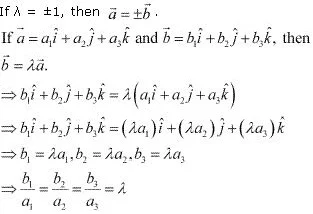Thus, the respective components of  a⃗ and b⃗ are proportional.
However, vectors  a⃗ and b⃗ can have different directions.
Hence, the statement given in D is incorrect.